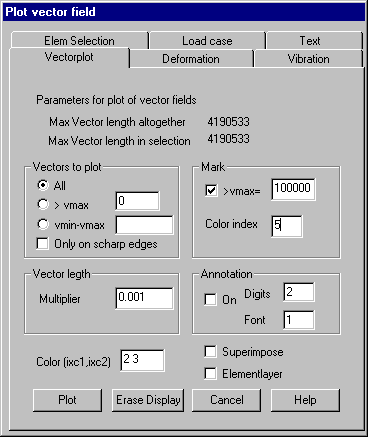Vector plot: Set parameters for following plots of vector fields

This property page sets parameters for plotting vector fields. Vectors are plotted as straight colored lines without arrows.

If the structure is displayed as a surface plot, only vectors belonging to visible nodes are plotted, however because of rounding errors the inquiry of visible nodes may not always be correct. If only sharp edges are plotted, alternatively the vectors in all nodes or only vectors on nodes of sharp edges may be plotted.

Following dialog shows the available options:Vectors to plot

All: All vectors of the active vector field are plotted for selected elements.

> max: Only those vectors are plotted whose length is greater than the given value.

vmin - vmax: Only those vectors are plotted whose length is within the specified range. The selection of vector length makes it possible to do several overlapping plots with colors appropriate to vector length.

Only on sharp edges: If this option is set, only vectors in nodes belonging to sharp edges are plotted.

Vector length

The plotted length of the largest defined vector must be given. Vectors are multiplied by the given factor.

Mark

This options makes it possible to emphasize vectors whose length exceeds a given value. The length and color index must be given.

Annotation

Optionally vectors can be annotated by the value of their length. The number of digits after decimal point and the font ID must be given.

Color

The color index used for plotting vectors must be given. In case of vector crosses 2 indices must be provided.

Superimpose

With this option set the plots are done within distinct layers. By this way vectors for different load cases can be plotted simultaneously using different colors.

Element layer

If this option is set and there are given layer IDs for different parts of the structure, the vectors are plotted in different layers, so that the vectors of different part of the structure may easily be shown or hidden.

The plot is done by pressing „Plot“. Button „Erase Display“ erases all display lists.